# Geometric Cliques

If you have `N` points in the plane what is the largest subset of those points such that each point is within a distance of `D` of all the others. It seems pretty innocuous right? Turns out it’s a great big beautiful disaster.

Here’s a example of `N = 10` points where the maximum subset are the points collected with dotted lines which are each within a distance `D` of each other. There is no bigger set.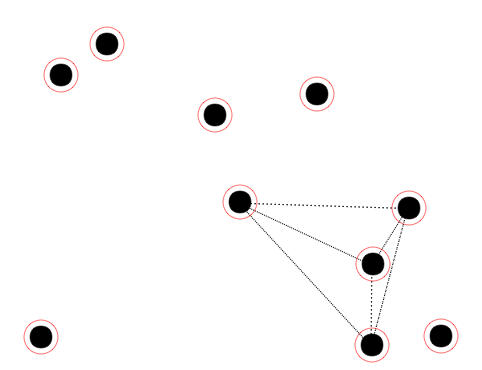Trying to compute every subset of points and checking if they are all within `D` of each other will take exponential time `O(2^N)`. So we need a better approach. Let’s try picking a point which will will assume to be part of this clique. Then all the candidate points that are not within `D` of that point won’t be in the clique. For example if we have three co-linear points spaced by `D` and select the middle one to build our clique then either the left points can be in the clique or the right one but not both. This does give us a heuristic. We can take each point filter out points that aren’t within `D` and then run a brute force search to find the maximal clique.

Let’s try and do better, as this could still be exponential depending on how the points are clustered. Let’s start with a bunch of points:

Pick any two points and assume that they are going to be the furthest points apart in our clique let this distance be `F`, so `F <= D`.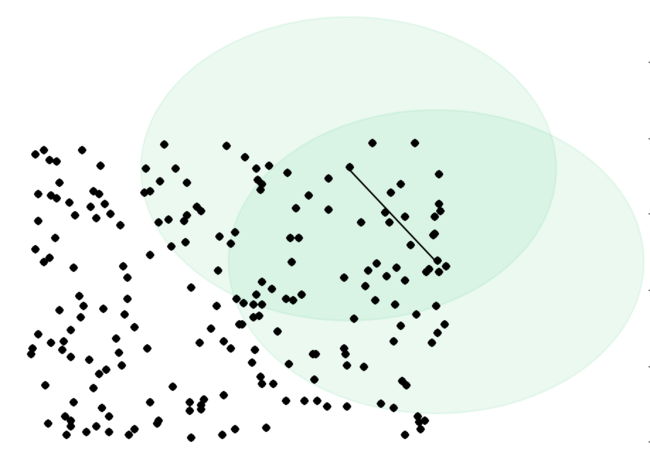If we filter out all points more than `F` from these two points we get this situation

``````# try all candidates for furthest points
for i in xrange(N):
for j in xrange(i + 1, N):
xi, yi = points[i]
xj, yj = points[j]

if distance(xi, yi, xj, yj) > D: continue

# Furthest pair in our clique
F = distance(xi, yi, xj, yj)

lens = []

for k in xrange(N):

if k == i: continue
if k == j: continue

xk, yk = points[k]

if distance(xi, yi, xk, yk) > F: continue
if distance(xj, yj, xk, yk) > F: continue

lens.append(points[k])
``````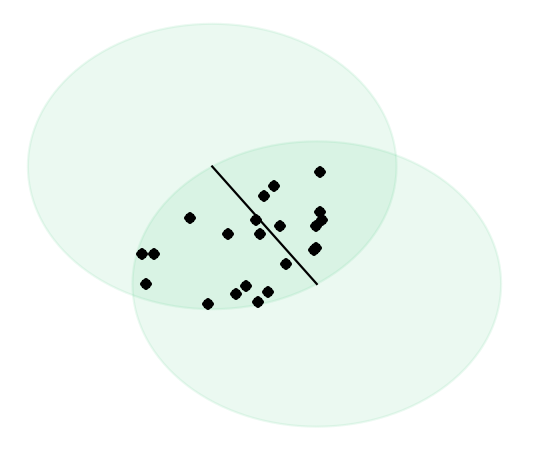Points inside the intersection of these two circles - the lens shape - are within `F` of both points at the end of the line and `F <= D`. I thought this way the end of the story. But we can’t simply select all of these points as a clique because they may not be within `D` of each other. For example the top and bottom points in the lens shape might be further than `D` apart so we need to do some more work.

First note that all the points above the dotted lines are within `F` and therefore `D` of each other so they are a potential clique, as are points below the dotted line. But there may be a bigger clique incorporating points from both sides of the lines. If we pick a certain point below the line for our clique we are forbidden from picking any points more than `D` away from that point. Incidentally these forbidden points will all lie on the other side of the line. Take a moment, look at the picture above and make sure you are happy with that.

Now lets separate the points inside the lens shape into two sets. Those above the dotted line and those below. This can be done buy taking the signed area of the triangle created between the two points at the end of the line and the point in question. If the area is positive the point is above the line and is it’s negative it’s below the line.

``````top, bot = [], []
M = len(lens)

for k in xrange(M):
xk, yk = lens[k]

if area((xi, yi), (xj, yj), (xk, yk)) >= 0:
top.append(lens[k])
else:
bot.append(lens[k])
``````

The situation is now like this: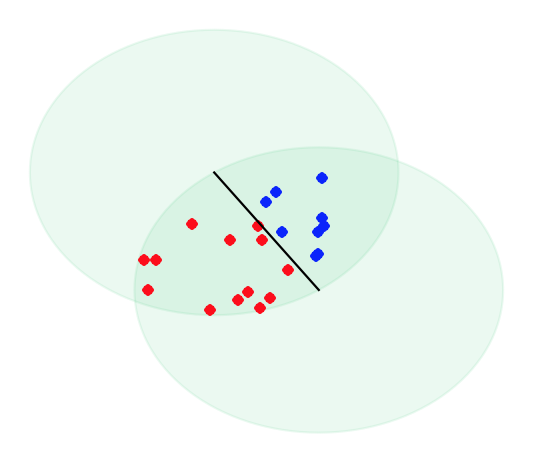Let’s now treat each point as a vertex and connect vertices in one set with vertices in the other if they are further than `D` away. Using fewer points so it’s not as cluttered the situation could look like this. Red lines denote points further than `D` apart and some point don’t have edges connected to them. This is fine, it just means all points are within `D` of them.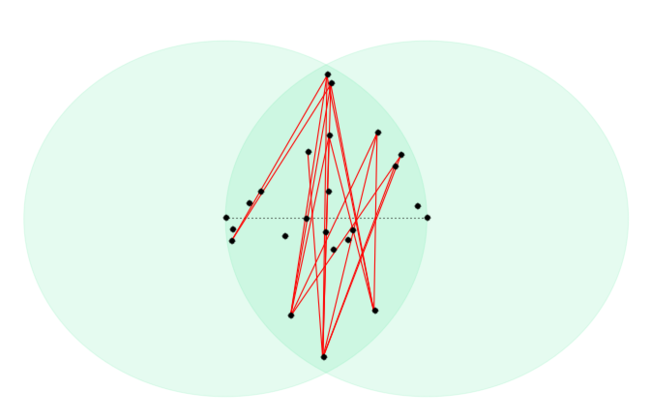We’ve now constructed a bipartite graph representing some of the geometric constraints we are interested in. So our task is to select the maximum number of points from this set such that we don’t have any two points connected by a red line because that means they are too far away. Vertices with no edges connected to them are freebies. They are within `D` of all of points so there’s no reason not to pick them.

Our problem of selecting the maximum number of vertices in a graph such that no two points have an incident edge is the problem of computing the maximum independent set of a graph. This is unfortunately NP-Complete in a general graph but in a bi-partite graph the number of vertices in the maximum independent set equals the number of edges in a minimum edge covering. This in turn is equal to the maximum-flow of the graph. This series of dualities is called König’s theorem.

Without the bi-partite structure, computing the maximum independent set is NP-Complete where as maximum-flow can be computed in a general graph in polynomial time. So we’re in much better shape. We compute the maximum flow of this graph and that value `f` plus the number of nodes without edges `top_set` and `bot_set` plus `2` for the end points of the line as our solution for that pair of points.

``````def max_bipartite_independent(top, bot):

graph = collections.defaultdict(dict)

top_set = set( [i for i in xrange( len(top)) ])
bot_set = set( [i for i in xrange( len(bot)) ])

for i in xrange(len(top)):
for j in xrange(len(bot)):

xi, yi = top[i]
xj, yj = bot[j]

if distance(xi, yi, xj, yj) > F:

node_i = 'TOP' + str(i)
node_j = 'BOT' + str(j)

src = 'SOURCE-NODE'
snk = 'SINK-NODE'

graph[node_i][node_j] = 1
graph[src][node_i] = MAX_INT
graph[node_j][snk] = MAX_INT

f = flow.max_flow(graph, 'SOURCE-NODE', 'SINK-NODE')
solution = f + len(top_set) + len(bot_set) + 2
return solution
``````

There are a few different algorithms to compute maximum flow . The following is a simple implementation of the Ford-Fulkerson algorithm using Dijkstra’s algorithm to find augmenting paths.

``````import Queue

def dijkstra(graph, source, sink):

q = Queue.PriorityQueue()
q.put((0, source, []))
visited = set([source])

while not q.empty():
length, node, path = q.get()

# Found a path return the capacity
if node == sink:
cap = None
for a, b in path:
if cap is None or graph[a][b] < cap:
cap = graph[a][b]
return cap, path

# Visit next node
for child in graph[node].keys():
if not child in visited and graph[node][child] > 0:
next_state = (length+1, child, path + [(node, child)])
q.put(next_state)

# No paths remaining
return None, None
``````

And the remaining code to compute the maximum-flow of the graph:

``````def max_flow(graph, source, sink):

flow = 0

while True:
capacity, path = dijkstra(graph, source, sink)
if not capacity: return flow

for a, b in path:
graph[a][b] = graph[a].get(b, 0) - capacity
graph[b][a] = graph[b].get(a, 0) + capacity
flow += capacity

return flow
``````

Cool so we’ve finally go all the pieces needed to solve this problem. We try every pair of points `O(N^2)` as the candidate for the two furtherest points in our clique then of the points the fall inside the lens we build a bipartite graph and compute the maximum independent set which corresponds to the maximum clique size.

All in the algorithm takes `O(V^2)` attempting each pair of candidates points. But with the maximum-flow inner loop it’s `O(V^5)`. This appears to be optimal without using a faster maximum matcher. Full source code is here, maximum flow code and a little visualizer.